# Compound Interest

What's compound interest and what's the formula for compound interest in Excel? This example gives you the answers to these questions.

1. Assume you put \$100 into a bank. How much will your investment be worth after 1 year at an annual interest rate of 8%? The answer is \$108.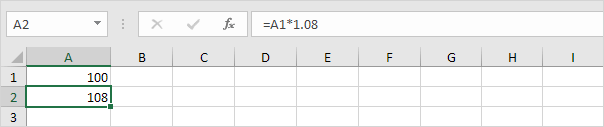2. Now this interest (\$8) will also earn interest (compound interest) next year. How much will your investment be worth after 2 years at an annual interest rate of 8%? The answer is \$116.64.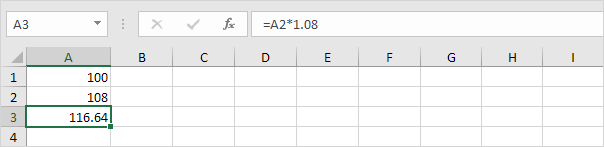3. How much will your investment be worth after 5 years? Simply drag the formula down to cell A6.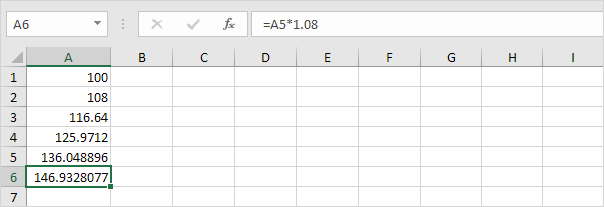4. All we did was multiplying 100 by 1.08, 5 times. So we can also directly calculate the value of the investment after 5 years.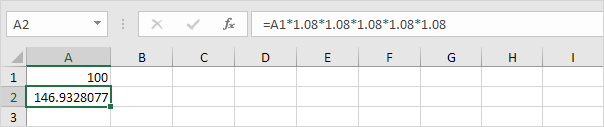which is the same as: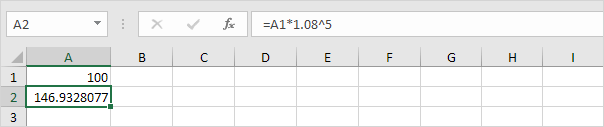Note: there is no special function for compound interest in Excel. However, you can easily create a compound interest calculator to compare different rates and different durations.

5. Assume you put \$100 into a bank. How much will your investment be worth after 5 years at an annual interest rate of 8%? You already know the answer.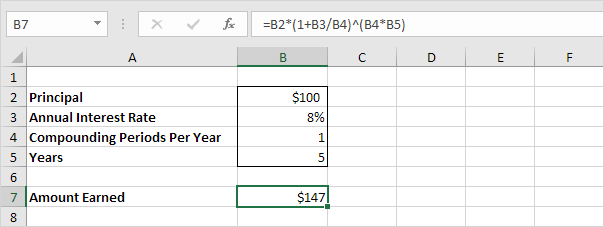Note: the compound interest formula reduces to =100*(1+0.08/1)^(1*5), =100*(1.08)^5

6. Assume you put \$10,000 into a bank. How much will your investment be worth after 15 years at an annual interest rate of 4% compounded quarterly? The answer is \$18,167.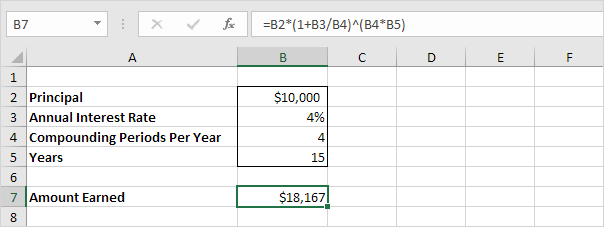Note: the compound interest formula reduces to =10000*(1+0.04/4)^(4*15), =10000*(1.01)^60

7. Assume you put \$10,000 into a bank. How much will your investment be worth after 10 years at an annual interest rate of 5% compounded monthly? The answer is \$16,470.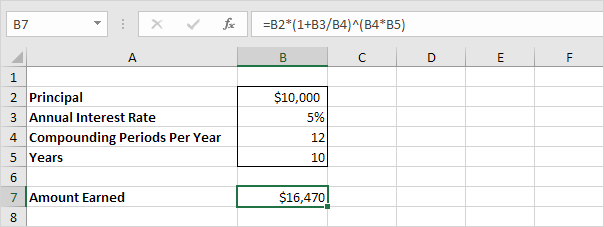Note: the compound interest formula always works. If you're interested, download the Excel file and try it yourself!

Go to Next Chapter: Statistical Functions